Courses

Test: Multiplication - 3

20 Questions MCQ Test Mathematics for Class 3: NCERT | Test: Multiplication - 3

Description
Attempt Test: Multiplication - 3 | 20 questions in 40 minutes | Mock test for Class 3 preparation | Free important questions MCQ to study Mathematics for Class 3: NCERT for Class 3 Exam | Download free PDF with solutions
QUESTION: 1

Solution:

845 x  = 845

QUESTION: 2

7 + 7 + 7 + 7 + 7 + 7 = □ x 7

Solution:

7 + 7 + 7 + 7 + 7 + 7 = 42
6 x 7 = 42

QUESTION: 3

Which of the following is the multiplication sentence for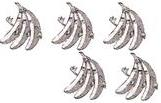Solution:

5 x 3 = 15

QUESTION: 4

17 x 5 = (10 x 5) + (D x 5)

Solution:

17 x 5 = (10 + 7) x 5 = 10 x 5 +  x 5

QUESTION: 5

There are 48 flowers in a garland. How many flowers are there in 8 garlands?

Solution:

48 x 8

QUESTION: 6

Radhika bought two burgers and a small coke. The burgers cost Rs. 24 each and the coke costs Rs.12. How much did her meal cost?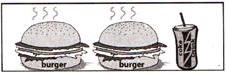Solution:

2 x 24 + 12 = 48 + 12 = Rs. 60

QUESTION: 7

Each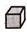weighs 2 kg. How much does the following figure weigh?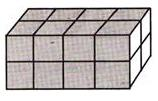Solution:

16 x 2 = 32 kg

QUESTION: 8

In the multiplication example shown, find the value of A?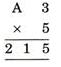Solution: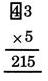QUESTION: 9

Sunitha discovered a multiplication pattern. She wrote the number sentences below to show the pattern?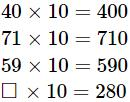What number should go in the box to complete the last number sentence?

Solution:

 x 10 = 280

QUESTION: 10

If the cost of 1 packet of PARLE-G biscuits is Rs. 5, how many packets can I get for Rs. 30 with the FREE OFFER shown?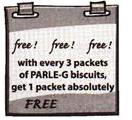Solution:

With Rs. 30, person can get 6 packets of Rs. 5 each, also with 3 packets you can get 1 packet free. so total he'll get

6 + 2 = 8 packets

QUESTION: 11

If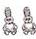stands for 20, then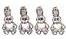will stand for:

Solution:

20 x 2 = 40

QUESTION: 12

If the product of all the three numbers in any row, column or diagonal is same, then find the missing term in the given square?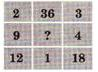Solution:

12 x 1 x 18 = 9 x  x 4

QUESTION: 13

Study the following diagram and then find the missing term.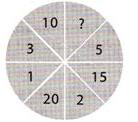Solution:

1 x 5 = 5, 2 x 5 = 10, 3 x 5 = 15,  x 5 = 20

QUESTION: 14

Ramu and Somu wrote two 2-digit numbers, each having the digit 1 only once and in the place shown.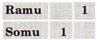If Ramu wrote the greatest two digit number and Somu wrote the least two digit number, then the product of the numbers is:

Solution:

91 x 10 = 910

QUESTION: 15

Find the missing term in the following?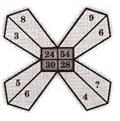Solution:

3 x 8 = 24, 9 x 6 = 54, 5 x 6 = 30, 4 x  = 28

QUESTION: 16

The total number of legs of 6 deers altogether = 6 times of_________: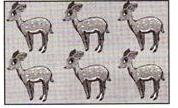Solution:

Since one deer has four legs. Number of legs of 6 deers are 6 times of 4.

QUESTION: 17

There are 19 books. Each book has 20 pages. How many pages are there in all?

Solution:

Number of pages in all = 19 x 20 = 380

QUESTION: 18

What is the missing number in last circle?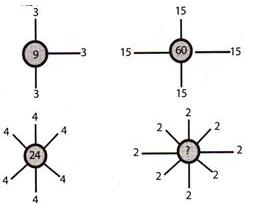Solution:

Number of 2?s are 8.
∴ Missing number = 2 x 8  = 16

QUESTION: 19

Sahil's mathematics teacher takes 6 days to complete a chapter. If everyday she uses three chalk pieces, then number of chalk pieces she used to complete such three chapters are: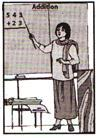Solution:

1 chapter takes 6 days.
∴ 3 chapters will take.
(6×3) =18 days.
Number of chalk pieces used in 1 day = 3
∴ Number of chalk pieces used in 18 days = 18 x 3 = 54

QUESTION: 20

What is the missing term?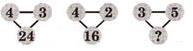Solution: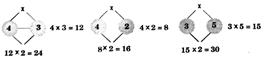Use Code STAYHOME200 and get INR 200 additional OFF Use Coupon Code

Track your progress, build streaks, highlight & save important lessons and more!

Similar ContentRelated tests